Home Puzzles Solvers Archives Competitions Techniques News Contact Puzzle Packs Publication Crosswords Codewords
Competitions 
 Sudoku Weekly Competition Advance puzzles are now availableNow available for your iPhone Cool Sudoku Visit our site to see the great iPhone application that brings you Sudoku, Killer, Jigsaw, Kakuro and Mini Sudoku to your iPhone/iPod Touch. We are running Daily Competitions and will be supporting a leader board for each puzzle type. Click to go to the Cool Sudoku Site or use the Button below to view in iTunes#818, May 22 to May 28: Extreme Sudoku Puzzle WARNING: You are on the Weekly 'Extreme' Sudoku Sudoku Page. If you insist on continuing, print out the Sudoku from the link below and when you have completed it, enter the numbers in the box on the form with your name and email address. Correct entries contribute to your personal history in Sudoku puzzles and your score and rank. The deadline is Saturday night, any time up to midnight. All Extreme puzzles have a single solution and at least one logical solve path, although this might be obscure to say the least. Please NOTE: There is now a Sudoku Solver available - click HERE
 3 9 7 2 3 5 5 7 7 8 1 5 2 7 5 6 1 2 9 3 8 1 8 5 1 2 7 8
EXTREME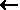Type in blank cells!!Prefer the old system?
Click on the button below

Popups must be enabled

> Copy and Paste <

 Time Taken Do let us know how long this took or give us your own rating

Country *
Tick this box to save details in a cookie

## Last weeks's Solution: Extreme no. 817

 9 7 1 6 2 3 8 5 4 4 5 8 7 9 1 3 6 2 6 2 3 8 5 4 9 7 1 8 9 7 1 4 2 5 3 6 1 3 6 5 8 9 4 2 7 2 4 5 3 6 7 1 8 9 3 6 4 9 7 5 2 1 8 5 8 9 2 1 6 7 4 3 7 1 2 4 3 8 6 9 5

## Archive

Number 1: Puzzle - Solution
Number 2: Puzzle - Solution
Number 3: Puzzle - Solution
Number 4: Puzzle - Solution
Number 5: Puzzle - Solution
Number 6: Puzzle - Solution
Number 7: Puzzle - Solution
Number 8: Puzzle - Solution
Number 9: Puzzle - Solution
Number 10: Puzzle - Solution
Number 11: Puzzle - Solution
Number 12: Puzzle - Solution
Number 13: Puzzle - Solution
Number 14: Puzzle - Solution
Number 15: Puzzle - Solution
Number 16: Puzzle - Solution
Number 17: Puzzle - Solution
Number 18: Puzzle - Solution
Number 19: Puzzle - Solution
Number 20: Puzzle - Solution
Number 21: Puzzle - Solution
Number 22: Puzzle - Solution
Number 23: Puzzle - Solution
Number 24: Puzzle - Solution
Number 25: Puzzle - Solution
Number 26: Puzzle - Solution
Number 27: Puzzle - Solution
Number 28: Puzzle - Solution
Number 29: Puzzle - Solution
Number 30: Puzzle - Solution
Number 31: Puzzle - Solution
Number 32: Puzzle - Solution
Number 33: Puzzle - Solution
Number 34: Puzzle - Solution
Number 35: Puzzle - Solution
Number 36: Puzzle - Solution
Number 37: Puzzle - Solution
Number 38: Puzzle - Solution
Number 39: Puzzle - Solution
Number 40: Puzzle - Solution
Number 41: Puzzle - Solution
Number 42: Puzzle - Solution
Number 43: Puzzle - Solution
Number 44: Puzzle - Solution
Number 45: Puzzle - Solution
Number 46: Puzzle - Solution
Number 47: Puzzle - Solution
Number 48: Puzzle - Solution
Number 49: Puzzle - Solution
Number 50: Puzzle - Solution
Number 51: Puzzle - Solution
Number 52: Puzzle - Solution
Number 53: Puzzle - Solution
Number 54: Puzzle - Solution
Number 55: Puzzle - Solution
Number 56: Puzzle - Solution
Number 57: Puzzle - Solution
Number 58: Puzzle - Solution
Number 59: Puzzle - Solution
Number 60: Puzzle - Solution
Number 61: Puzzle - Solution
Number 62: Puzzle - Solution
Number 63: Puzzle - Solution
Number 64: Puzzle - Solution
Number 65: Puzzle - Solution
Number 66: Puzzle - Solution
Number 67: Puzzle - Solution
Number 68: Puzzle - Solution
Number 69: Puzzle - Solution
Number 70: Puzzle - Solution
Number 71: Puzzle - Solution
Number 72: Puzzle - Solution
Number 73: Puzzle - Solution
Number 74: Puzzle - Solution
Number 75: Puzzle - Solution
Number 76: Puzzle - Solution
Number 77: Puzzle - Solution
Number 78: Puzzle - Solution
Number 79: Puzzle - Solution
Number 80: Puzzle - Solution
Number 81: Puzzle - Solution
Number 82: Puzzle - Solution
Number 83: Puzzle - Solution
Number 84: Puzzle - Solution
Number 85: Puzzle - Solution
Number 86: Puzzle - Solution
Number 87: Puzzle - Solution
Number 88: Puzzle - Solution
Number 89: Puzzle - Solution
Number 90: Puzzle - Solution
Number 91: Puzzle - Solution
Number 92: Puzzle - Solution
Number 93: Puzzle - Solution
Number 94: Puzzle - Solution
Number 95: Puzzle - Solution
Number 96: Puzzle - Solution
Number 97: Puzzle - Solution
Number 98: Puzzle - Solution
Number 99: Puzzle - Solution
Number 100: Puzzle - Solution
Number 101: Puzzle - Solution
Number 102: Puzzle - Solution
Number 103: Puzzle - Solution
Number 104: Puzzle - Solution
Number 105: Puzzle - Solution
Number 106: Puzzle - Solution
Number 107: Puzzle - Solution
Number 108: Puzzle - Solution
Number 109: Puzzle - Solution
Number 110: Puzzle - Solution
Number 111: Puzzle - Solution
Number 112: Puzzle - Solution
Number 113: Puzzle - Solution
Number 114: Puzzle - Solution
Number 115: Puzzle - Solution
Number 116: Puzzle - Solution
Number 117: Puzzle - Solution
Number 118: Puzzle - Solution
Number 119: Puzzle - Solution
Number 120: Puzzle - Solution
Number 121: Puzzle - Solution
Number 122: Puzzle - Solution
Number 123: Puzzle - Solution
Number 124: Puzzle - Solution
Number 125: Puzzle - Solution
Number 126: Puzzle - Solution
Number 127: Puzzle - Solution
Number 128: Puzzle - Solution
Number 129: Puzzle - Solution
Number 130: Puzzle - Solution
Number 131: Puzzle - Solution
Number 132: Puzzle - Solution
Number 133: Puzzle - Solution
Number 134: Puzzle - Solution
Number 135: Puzzle - Solution
Number 136: Puzzle - Solution
Number 137: Puzzle - Solution
Number 138: Puzzle - Solution
Number 139: Puzzle - Solution
Number 140: Puzzle - Solution
Number 141: Puzzle - Solution
Number 142: Puzzle - Solution
Number 143: Puzzle - Solution
Number 144: Puzzle - Solution
Number 145: Puzzle - Solution
Number 146: Puzzle - Solution
Number 147: Puzzle - Solution
Number 148: Puzzle - Solution
Number 149: Puzzle - Solution
Number 150: Puzzle - Solution
Number 151: Puzzle - Solution
Number 152: Puzzle - Solution
Number 153: Puzzle - Solution
Number 154: Puzzle - Solution
Number 155: Puzzle - Solution
Number 156: Puzzle - Solution
Number 157: Puzzle - Solution
Number 158: Puzzle - Solution
Number 159: Puzzle - Solution
Number 160: Puzzle - Solution
Number 161: Puzzle - Solution
Number 162: Puzzle - Solution
Number 163: Puzzle - Solution
Number 164: Puzzle - Solution
Number 165: Puzzle - Solution
Number 166: Puzzle - Solution
Number 167: Puzzle - Solution
Number 168: Puzzle - Solution
Number 169: Puzzle - Solution
Number 170: Puzzle - Solution
Number 171: Puzzle - Solution
Number 172: Puzzle - Solution
Number 173: Puzzle - Solution
Number 174: Puzzle - Solution
Number 175: Puzzle - Solution
Number 176: Puzzle - Solution
Number 177: Puzzle - Solution
Number 178: Puzzle - Solution
Number 179: Puzzle - Solution
Number 180: Puzzle - Solution
Number 181: Puzzle - Solution
Number 182: Puzzle - Solution
Number 183: Puzzle - Solution
Number 184: Puzzle - Solution
Number 185: Puzzle - Solution
Number 186: Puzzle - Solution
Number 187: Puzzle - Solution
Number 188: Puzzle - Solution
Number 189: Puzzle - Solution
Number 190: Puzzle - Solution
Number 191: Puzzle - Solution
Number 192: Puzzle - Solution
Number 193: Puzzle - Solution
Number 194: Puzzle - Solution
Number 195: Puzzle - Solution
Number 196: Puzzle - Solution
Number 197: Puzzle - Solution
Number 198: Puzzle - Solution
Number 199: Puzzle - Solution
Number 200: Puzzle - Solution
Number 201: Puzzle - Solution
Number 202: Puzzle - Solution
Number 203: Puzzle - Solution
Number 204: Puzzle - Solution
Number 205: Puzzle - Solution
Number 206: Puzzle - Solution
Number 207: Puzzle - Solution
Number 208: Puzzle - Solution
Number 209: Puzzle - Solution
Number 210: Puzzle - Solution
Number 211: Puzzle - Solution
Number 212: Puzzle - Solution
Number 213: Puzzle - Solution
Number 214: Puzzle - Solution
Number 215: Puzzle - Solution
Number 216: Puzzle - Solution
Number 217: Puzzle - Solution
Number 218: Puzzle - Solution
Number 219: Puzzle - Solution
Number 220: Puzzle - Solution
Number 221: Puzzle - Solution
Number 222: Puzzle - Solution
Number 223: Puzzle - Solution
Number 224: Puzzle - Solution
Number 225: Puzzle - Solution
Number 226: Puzzle - Solution
Number 227: Puzzle - Solution
Number 228: Puzzle - Solution
Number 229: Puzzle - Solution
Number 230: Puzzle - Solution
Number 231: Puzzle - Solution
Number 232: Puzzle - Solution
Number 233: Puzzle - Solution
Number 234: Puzzle - Solution
Number 235: Puzzle - Solution
Number 236: Puzzle - Solution
Number 237: Puzzle - Solution
Number 238: Puzzle - Solution
Number 239: Puzzle - Solution
Number 240: Puzzle - Solution
Number 241: Puzzle - Solution
Number 242: Puzzle - Solution
Number 243: Puzzle - Solution
Number 244: Puzzle - Solution
Number 245: Puzzle - Solution
Number 246: Puzzle - Solution
Number 247: Puzzle - Solution
Number 248: Puzzle - Solution
Number 249: Puzzle - Solution
Number 250: Puzzle - Solution
Number 251: Puzzle - Solution
Number 252: Puzzle - Solution
Number 253: Puzzle - Solution
Number 254: Puzzle - Solution
Number 255: Puzzle - Solution
Number 256: Puzzle - Solution
Number 257: Puzzle - Solution
Number 258: Puzzle - Solution
Number 259: Puzzle - Solution
Number 260: Puzzle - Solution
Number 261: Puzzle - Solution
Number 262: Puzzle - Solution
Number 263: Puzzle - Solution
Number 264: Puzzle - Solution
Number 265: Puzzle - Solution
Number 266: Puzzle - Solution
Number 267: Puzzle - Solution
Number 268: Puzzle - Solution
Number 269: Puzzle - Solution
Number 270: Puzzle - Solution
Number 271: Puzzle - Solution
Number 272: Puzzle - Solution
Number 273: Puzzle - Solution
Number 274: Puzzle - Solution
Number 275: Puzzle - Solution
Number 276: Puzzle - Solution
Number 277: Puzzle - Solution
Number 278: Puzzle - Solution
Number 279: Puzzle - Solution
Number 280: Puzzle - Solution
Number 281: Puzzle - Solution
Number 282: Puzzle - Solution
Number 283: Puzzle - Solution
Number 284: Puzzle - Solution
Number 285: Puzzle - Solution
Number 286: Puzzle - Solution
Number 287: Puzzle - Solution
Number 288: Puzzle - Solution
Number 289: Puzzle - Solution
Number 290: Puzzle - Solution
Number 291: Puzzle - Solution
Number 292: Puzzle - Solution
Number 293: Puzzle - Solution
Number 294: Puzzle - Solution
Number 295: Puzzle - Solution
Number 296: Puzzle - Solution
Number 297: Puzzle - Solution
Number 298: Puzzle - Solution
Number 299: Puzzle - Solution
Number 300: Puzzle - Solution
Number 301: Puzzle - Solution
Number 302: Puzzle - Solution
Number 303: Puzzle - Solution
Number 304: Puzzle - Solution
Number 305: Puzzle - Solution
Number 306: Puzzle - Solution
Number 307: Puzzle - Solution
Number 308: Puzzle - Solution
Number 309: Puzzle - Solution
Number 310: Puzzle - Solution
Number 311: Puzzle - Solution
Number 312: Puzzle - Solution
Number 313: Puzzle - Solution
Number 314: Puzzle - Solution
Number 315: Puzzle - Solution
Number 316: Puzzle - Solution
Number 317: Puzzle - Solution
Number 318: Puzzle - Solution
Number 319: Puzzle - Solution
Number 320: Puzzle - Solution
Number 321: Puzzle - Solution
Number 322: Puzzle - Solution
Number 323: Puzzle - Solution
Number 324: Puzzle - Solution
Number 325: Puzzle - Solution
Number 326: Puzzle - Solution
Number 327: Puzzle - Solution
Number 328: Puzzle - Solution
Number 329: Puzzle - Solution
Number 330: Puzzle - Solution
Number 331: Puzzle - Solution
Number 332: Puzzle - Solution
Number 333: Puzzle - Solution
Number 334: Puzzle - Solution
Number 335: Puzzle - Solution
Number 336: Puzzle - Solution
Number 337: Puzzle - Solution
Number 338: Puzzle - Solution
Number 339: Puzzle - Solution
Number 340: Puzzle - Solution
Number 341: Puzzle - Solution
Number 342: Puzzle - Solution
Number 343: Puzzle - Solution
Number 344: Puzzle - Solution
Number 345: Puzzle - Solution
Number 346: Puzzle - Solution
Number 347: Puzzle - Solution
Number 348: Puzzle - Solution
Number 349: Puzzle - Solution
Number 350: Puzzle - Solution
Number 351: Puzzle - Solution
Number 352: Puzzle - Solution
Number 353: Puzzle - Solution
Number 354: Puzzle - Solution
Number 355: Puzzle - Solution
Number 356: Puzzle - Solution
Number 357: Puzzle - Solution
Number 358: Puzzle - Solution
Number 359: Puzzle - Solution
Number 360: Puzzle - Solution
Number 361: Puzzle - Solution
Number 362: Puzzle - Solution
Number 363: Puzzle - Solution
Number 364: Puzzle - Solution
Number 365: Puzzle - Solution
Number 366: Puzzle - Solution
Number 367: Puzzle - Solution
Number 368: Puzzle - Solution
Number 369: Puzzle - Solution
Number 370: Puzzle - Solution
Number 371: Puzzle - Solution
Number 372: Puzzle - Solution
Number 373: Puzzle - Solution
Number 374: Puzzle - Solution
Number 375: Puzzle - Solution
Number 376: Puzzle - Solution
Number 377: Puzzle - Solution
Number 378: Puzzle - Solution
Number 379: Puzzle - Solution
Number 380: Puzzle - Solution
Number 381: Puzzle - Solution
Number 382: Puzzle - Solution
Number 383: Puzzle - Solution
Number 384: Puzzle - Solution
Number 385: Puzzle - Solution
Number 386: Puzzle - Solution
Number 387: Puzzle - Solution
Number 388: Puzzle - Solution
Number 389: Puzzle - Solution
Number 390: Puzzle - Solution
Number 391: Puzzle - Solution
Number 392: Puzzle - Solution
Number 393: Puzzle - Solution
Number 394: Puzzle - Solution
Number 395: Puzzle - Solution
Number 396: Puzzle - Solution
Number 397: Puzzle - Solution
Number 398: Puzzle - Solution
Number 399: Puzzle - Solution
Number 400: Puzzle - Solution
Number 401: Puzzle - Solution
Number 402: Puzzle - Solution
Number 403: Puzzle - Solution
Number 404: Puzzle - Solution
Number 405: Puzzle - Solution
Number 406: Puzzle - Solution
Number 407: Puzzle - Solution
Number 408: Puzzle - Solution
Number 409: Puzzle - Solution
Number 410: Puzzle - Solution
Number 411: Puzzle - Solution
Number 412: Puzzle - Solution
Number 413: Puzzle - Solution
Number 414: Puzzle - Solution
Number 415: Puzzle - Solution
Number 416: Puzzle - Solution
Number 417: Puzzle - Solution
Number 418: Puzzle - Solution
Number 419: Puzzle - Solution
Number 420: Puzzle - Solution
Number 421: Puzzle - Solution
Number 422: Puzzle - Solution
Number 423: Puzzle - Solution
Number 424: Puzzle - Solution
Number 425: Puzzle - Solution
Number 426: Puzzle - Solution
Number 427: Puzzle - Solution
Number 428: Puzzle - Solution
Number 429: Puzzle - Solution
Number 430: Puzzle - Solution
Number 431: Puzzle - Solution
Number 432: Puzzle - Solution
Number 433: Puzzle - Solution
Number 434: Puzzle - Solution
Number 435: Puzzle - Solution
Number 436: Puzzle - Solution
Number 437: Puzzle - Solution
Number 438: Puzzle - Solution
Number 439: Puzzle - Solution
Number 440: Puzzle - Solution
Number 441: Puzzle - Solution
Number 442: Puzzle - Solution
Number 443: Puzzle - Solution
Number 444: Puzzle - Solution
Number 445: Puzzle - Solution
Number 446: Puzzle - Solution
Number 447: Puzzle - Solution
Number 448: Puzzle - Solution
Number 449: Puzzle - Solution
Number 450: Puzzle - Solution
Number 451: Puzzle - Solution
Number 452: Puzzle - Solution
Number 453: Puzzle - Solution
Number 454: Puzzle - Solution
Number 455: Puzzle - Solution
Number 456: Puzzle - Solution
Number 457: Puzzle - Solution
Number 458: Puzzle - Solution
Number 459: Puzzle - Solution
Number 460: Puzzle - Solution
Number 461: Puzzle - Solution
Number 462: Puzzle - Solution
Number 463: Puzzle - Solution
Number 464: Puzzle - Solution
Number 465: Puzzle - Solution
Number 466: Puzzle - Solution
Number 467: Puzzle - Solution
Number 468: Puzzle - Solution
Number 469: Puzzle - Solution
Number 470: Puzzle - Solution
Number 471: Puzzle - Solution
Number 472: Puzzle - Solution
Number 473: Puzzle - Solution
Number 474: Puzzle - Solution
Number 475: Puzzle - Solution
Number 476: Puzzle - Solution
Number 477: Puzzle - Solution
Number 478: Puzzle - Solution
Number 479: Puzzle - Solution
Number 480: Puzzle - Solution
Number 481: Puzzle - Solution
Number 482: Puzzle - Solution
Number 483: Puzzle - Solution
Number 484: Puzzle - Solution
Number 485: Puzzle - Solution
Number 486: Puzzle - Solution
Number 487: Puzzle - Solution
Number 488: Puzzle - Solution
Number 489: Puzzle - Solution
Number 490: Puzzle - Solution
Number 491: Puzzle - Solution
Number 492: Puzzle - Solution
Number 493: Puzzle - Solution
Number 494: Puzzle - Solution
Number 495: Puzzle - Solution
Number 496: Puzzle - Solution
Number 497: Puzzle - Solution
Number 498: Puzzle - Solution
Number 499: Puzzle - Solution
Number 500: Puzzle - Solution
Number 501: Puzzle - Solution
Number 502: Puzzle - Solution
Number 503: Puzzle - Solution
Number 504: Puzzle - Solution
Number 505: Puzzle - Solution
Number 506: Puzzle - Solution
Number 507: Puzzle - Solution
Number 508: Puzzle - Solution
Number 509: Puzzle - Solution
Number 510: Puzzle - Solution
Number 511: Puzzle - Solution
Number 512: Puzzle - Solution
Number 513: Puzzle - Solution
Number 514: Puzzle - Solution
Number 515: Puzzle - Solution
Number 516: Puzzle - Solution
Number 517: Puzzle - Solution
Number 518: Puzzle - Solution
Number 519: Puzzle - Solution
Number 520: Puzzle - Solution
Number 521: Puzzle - Solution
Number 522: Puzzle - Solution
Number 523: Puzzle - Solution
Number 524: Puzzle - Solution
Number 525: Puzzle - Solution
Number 526: Puzzle - Solution
Number 527: Puzzle - Solution
Number 528: Puzzle - Solution
Number 529: Puzzle - Solution
Number 530: Puzzle - Solution
Number 531: Puzzle - Solution
Number 532: Puzzle - Solution
Number 533: Puzzle - Solution
Number 534: Puzzle - Solution
Number 535: Puzzle - Solution
Number 536: Puzzle - Solution
Number 537: Puzzle - Solution
Number 538: Puzzle - Solution
Number 539: Puzzle - Solution
Number 540: Puzzle - Solution
Number 541: Puzzle - Solution
Number 542: Puzzle - Solution
Number 543: Puzzle - Solution
Number 544: Puzzle - Solution
Number 545: Puzzle - Solution
Number 546: Puzzle - Solution
Number 547: Puzzle - Solution
Number 548: Puzzle - Solution
Number 549: Puzzle - Solution
Number 550: Puzzle - Solution
Number 551: Puzzle - Solution
Number 552: Puzzle - Solution
Number 553: Puzzle - Solution
Number 554: Puzzle - Solution
Number 555: Puzzle - Solution
Number 556: Puzzle - Solution
Number 557: Puzzle - Solution
Number 558: Puzzle - Solution
Number 559: Puzzle - Solution
Number 560: Puzzle - Solution
Number 561: Puzzle - Solution
Number 562: Puzzle - Solution
Number 563: Puzzle - Solution
Number 564: Puzzle - Solution
Number 565: Puzzle - Solution
Number 566: Puzzle - Solution
Number 567: Puzzle - Solution
Number 568: Puzzle - Solution
Number 569: Puzzle - Solution
Number 570: Puzzle - Solution
Number 571: Puzzle - Solution
Number 572: Puzzle - Solution
Number 573: Puzzle - Solution
Number 574: Puzzle - Solution
Number 575: Puzzle - Solution
Number 576: Puzzle - Solution
Number 577: Puzzle - Solution
Number 578: Puzzle - Solution
Number 579: Puzzle - Solution
Number 580: Puzzle - Solution
Number 581: Puzzle - Solution
Number 582: Puzzle - Solution
Number 583: Puzzle - Solution
Number 584: Puzzle - Solution
Number 585: Puzzle - Solution
Number 586: Puzzle - Solution
Number 587: Puzzle - Solution
Number 588: Puzzle - Solution
Number 589: Puzzle - Solution
Number 590: Puzzle - Solution
Number 591: Puzzle - Solution
Number 592: Puzzle - Solution
Number 593: Puzzle - Solution
Number 594: Puzzle - Solution
Number 595: Puzzle - Solution
Number 596: Puzzle - Solution
Number 597: Puzzle - Solution
Number 598: Puzzle - Solution
Number 599: Puzzle - Solution
Number 600: Puzzle - Solution
Number 601: Puzzle - Solution
Number 602: Puzzle - Solution
Number 603: Puzzle - Solution
Number 604: Puzzle - Solution
Number 605: Puzzle - Solution
Number 606: Puzzle - Solution
Number 607: Puzzle - Solution
Number 608: Puzzle - Solution
Number 609: Puzzle - Solution
Number 610: Puzzle - Solution
Number 611: Puzzle - Solution
Number 612: Puzzle - Solution
Number 613: Puzzle - Solution
Number 614: Puzzle - Solution
Number 615: Puzzle - Solution
Number 616: Puzzle - Solution
Number 617: Puzzle - Solution
Number 618: Puzzle - Solution
Number 619: Puzzle - Solution
Number 620: Puzzle - Solution
Number 621: Puzzle - Solution
Number 622: Puzzle - Solution
Number 623: Puzzle - Solution
Number 624: Puzzle - Solution
Number 625: Puzzle - Solution
Number 626: Puzzle - Solution
Number 627: Puzzle - Solution
Number 628: Puzzle - Solution
Number 629: Puzzle - Solution
Number 630: Puzzle - Solution
Number 631: Puzzle - Solution
Number 632: Puzzle - Solution
Number 633: Puzzle - Solution
Number 634: Puzzle - Solution
Number 635: Puzzle - Solution
Number 636: Puzzle - Solution
Number 637: Puzzle - Solution
Number 638: Puzzle - Solution
Number 639: Puzzle - Solution
Number 640: Puzzle - Solution
Number 641: Puzzle - Solution
Number 642: Puzzle - Solution
Number 643: Puzzle - Solution
Number 644: Puzzle - Solution
Number 645: Puzzle - Solution
Number 646: Puzzle - Solution
Number 647: Puzzle - Solution
Number 648: Puzzle - Solution
Number 649: Puzzle - Solution
Number 650: Puzzle - Solution
Number 651: Puzzle - Solution
Number 652: Puzzle - Solution
Number 653: Puzzle - Solution
Number 654: Puzzle - Solution
Number 655: Puzzle - Solution
Number 656: Puzzle - Solution
Number 657: Puzzle - Solution
Number 658: Puzzle - Solution
Number 659: Puzzle - Solution
Number 660: Puzzle - Solution
Number 661: Puzzle - Solution
Number 662: Puzzle - Solution
Number 663: Puzzle - Solution
Number 664: Puzzle - Solution
Number 665: Puzzle - Solution
Number 666: Puzzle - Solution
Number 667: Puzzle - Solution
Number 668: Puzzle - Solution
Number 669: Puzzle - Solution
Number 670: Puzzle - Solution
Number 671: Puzzle - Solution
Number 672: Puzzle - Solution
Number 673: Puzzle - Solution
Number 674: Puzzle - Solution
Number 675: Puzzle - Solution
Number 676: Puzzle - Solution
Number 677: Puzzle - Solution
Number 678: Puzzle - Solution
Number 679: Puzzle - Solution
Number 680: Puzzle - Solution
Number 681: Puzzle - Solution
Number 682: Puzzle - Solution
Number 683: Puzzle - Solution
Number 684: Puzzle - Solution
Number 685: Puzzle - Solution
Number 686: Puzzle - Solution
Number 687: Puzzle - Solution
Number 688: Puzzle - Solution
Number 689: Puzzle - Solution
Number 690: Puzzle - Solution
Number 691: Puzzle - Solution
Number 692: Puzzle - Solution
Number 693: Puzzle - Solution
Number 694: Puzzle - Solution
Number 695: Puzzle - Solution
Number 696: Puzzle - Solution
Number 697: Puzzle - Solution
Number 698: Puzzle - Solution
Number 699: Puzzle - Solution
Number 700: Puzzle - Solution
Number 701: Puzzle - Solution
Number 702: Puzzle - Solution
Number 703: Puzzle - Solution
Number 704: Puzzle - Solution
Number 705: Puzzle - Solution
Number 706: Puzzle - Solution
Number 707: Puzzle - Solution
Number 708: Puzzle - Solution
Number 709: Puzzle - Solution
Number 710: Puzzle - Solution
Number 711: Puzzle - Solution
Number 712: Puzzle - Solution
Number 713: Puzzle - Solution
Number 714: Puzzle - Solution
Number 715: Puzzle - Solution
Number 716: Puzzle - Solution
Number 717: Puzzle - Solution
Number 718: Puzzle - Solution
Number 719: Puzzle - Solution
Number 720: Puzzle - Solution
Number 721: Puzzle - Solution
Number 722: Puzzle - Solution
Number 723: Puzzle - Solution
Number 724: Puzzle - Solution
Number 725: Puzzle - Solution
Number 726: Puzzle - Solution
Number 727: Puzzle - Solution
Number 728: Puzzle - Solution
Number 729: Puzzle - Solution
Number 730: Puzzle - Solution
Number 731: Puzzle - Solution
Number 732: Puzzle - Solution
Number 733: Puzzle - Solution
Number 734: Puzzle - Solution
Number 735: Puzzle - Solution
Number 736: Puzzle - Solution
Number 737: Puzzle - Solution
Number 738: Puzzle - Solution
Number 739: Puzzle - Solution
Number 740: Puzzle - Solution
Number 741: Puzzle - Solution
Number 742: Puzzle - Solution
Number 743: Puzzle - Solution
Number 744: Puzzle - Solution
Number 745: Puzzle - Solution
Number 746: Puzzle - Solution
Number 747: Puzzle - Solution
Number 748: Puzzle - Solution
Number 749: Puzzle - Solution
Number 750: Puzzle - Solution
Number 751: Puzzle - Solution
Number 752: Puzzle - Solution
Number 753: Puzzle - Solution
Number 754: Puzzle - Solution
Number 755: Puzzle - Solution
Number 756: Puzzle - Solution
Number 757: Puzzle - Solution
Number 758: Puzzle - Solution
Number 759: Puzzle - Solution
Number 760: Puzzle - Solution
Number 761: Puzzle - Solution
Number 762: Puzzle - Solution
Number 763: Puzzle - Solution
Number 764: Puzzle - Solution
Number 765: Puzzle - Solution
Number 766: Puzzle - Solution
Number 767: Puzzle - Solution
Number 768: Puzzle - Solution
Number 769: Puzzle - Solution
Number 770: Puzzle - Solution
Number 771: Puzzle - Solution
Number 772: Puzzle - Solution
Number 773: Puzzle - Solution
Number 774: Puzzle - Solution
Number 775: Puzzle - Solution
Number 776: Puzzle - Solution
Number 777: Puzzle - Solution
Number 778: Puzzle - Solution
Number 779: Puzzle - Solution
Number 780: Puzzle - Solution
Number 781: Puzzle - Solution
Number 782: Puzzle - Solution
Number 783: Puzzle - Solution
Number 784: Puzzle - Solution
Number 785: Puzzle - Solution
Number 786: Puzzle - Solution
Number 787: Puzzle - Solution
Number 788: Puzzle - Solution
Number 789: Puzzle - Solution
Number 790: Puzzle - Solution
Number 791: Puzzle - Solution
Number 792: Puzzle - Solution
Number 793: Puzzle - Solution
Number 794: Puzzle - Solution
Number 795: Puzzle - Solution
Number 796: Puzzle - Solution
Number 797: Puzzle - Solution
Number 798: Puzzle - Solution
Number 799: Puzzle - Solution
Number 800: Puzzle - Solution
Number 801: Puzzle - Solution
Number 802: Puzzle - Solution
Number 803: Puzzle - Solution
Number 804: Puzzle - Solution
Number 805: Puzzle - Solution
Number 806: Puzzle - Solution
Number 807: Puzzle - Solution
Number 808: Puzzle - Solution
Number 809: Puzzle - Solution
Number 810: Puzzle - Solution
Number 811: Puzzle - Solution
Number 812: Puzzle - Solution
Number 813: Puzzle - Solution
Number 814: Puzzle - Solution
Number 815: Puzzle - Solution
Number 816: Puzzle - Solution
Number 817: Puzzle - Solution
Number 818: Puzzle -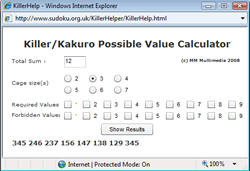Need help with your Puzzle solving In addition to our solvers we also offer utilities to help you solve various puzzles. One example is out Killer/Kakuro possible value calculator.

Extreme Sudoku | Jigsaw | KillerOnline SUDOKU - Play Sudoku Online NOW. All grades available.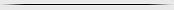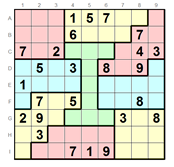Play Jigsaw Sudoku OnlinePlay Killer Sudoku Online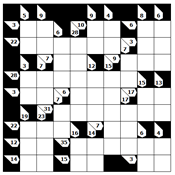Play Kakuro Online